# The most important invariants in basic electronics

The two basic laws in circuit analysis, Kirchhoffs Voltage and Current Laws,

1. [KVL] Voltage across the same pair of points is the same no matter what paths you take
2. [KCL] Current stay the same along the same path

are often taught in basic circuit analysis, but most of the time, they taught it in the context of nodal analysis, which you have a little more complicated meshes with multiple theoretical power source (voltage or current) that simple series/parallel circuit rules are not enough to solve the puzzle.

However, these two fundamental concepts are useful to develop insights that help you estimate quantities in a circuit quickly like a pro.

Kirchhoffs Voltage Law [KVL] can be applied to a parallel circuit of 2 branches (often the case when measuring additional loading effect). Let say the two branches are applied (loaded) at a voltage output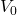, whichmight change depending on the branches (loading).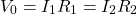You can exploit the algebra to quickly calculate the current of any branch without first computing the overall resistance or current: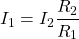Kirchhoffs Current Law [KCL] is useful in analyzing energy loss over resistance in wires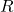. For example, in high school physics, we discuss why we have high voltage power lines for bulk energy transmission despite it’s more dangerous. The traditional explanation is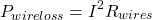so the lower the current is (which can be done through stepping up the voltage, traditionally done with AC signal through transformers, to maintain the same power). But how about other form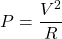Technically, it’s possible, but you have to be very careful that the voltage we are talking about is across the wire with resistive losses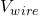, NOT the load voltage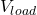.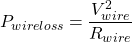changes depending on the output load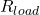, so you have to derive the assuming an arbitrary, which will happen to cancel itself out and end up the same as if you think of everything in terms of current first:So the bottom line is that most of the time, it is easier to think in terms of current in most circuit analysis because current won’t change along the same path. This is especially true when your problem has varying impedances/load which will disturb the voltage.

Of course, if the problem screams direct application using KVL, don’t go all the way converting it back to current. You will find the current-first approach useful when we get to semiconductors like diode, voltage references, BJTs,.

I usually think of voltage as a consequence or effect of current flushing into a transducer (e.g. resistor), so it’s subjected to change and therefore messy to use when solving circuit puzzles. Solving circuit analysis problems are often an exercise of identifying invariants and inferring the remaining quantities.

143 total views,  1 views today

Subscribe
Notify of